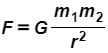Start typing, then use the up and down arrows to select an option from the list.
# Newton’s Law of Gravitation describes the attraction force between two masses. The equation is,where F is in [ kg·m / s2], m1 and m2 are masses in [ kg], and r is the distance in [ m] between them.Determine the units of the Universal Constant G.